## 【预测模型】基于matlab BP神经网络短时交通流预测【含Matlab源码 687期】

el/2023/6/3 15:37:37

## 一、简介

1 概述
BP（Back Propagation）神经网络是1986年由Rumelhart和McCelland为首的科研小组提出，参见他们发表在Nature上的论文 Learning representations by back-propagating errors 。

BP神经网络是一种按误差逆传播算法训练的多层前馈网络，是目前应用最广泛的神经网络模型之一。BP网络能学习和存贮大量的 输入-输出模式映射关系，而无需事前揭示描述这种映射关系的数学方程。它的学习规则是使用最速下降法，通过反向传播来不断 调整网络的权值和阈值，使网络的误差平方和最小。

2 BP算法的基本思想

BP算法的信号流向图如下图所示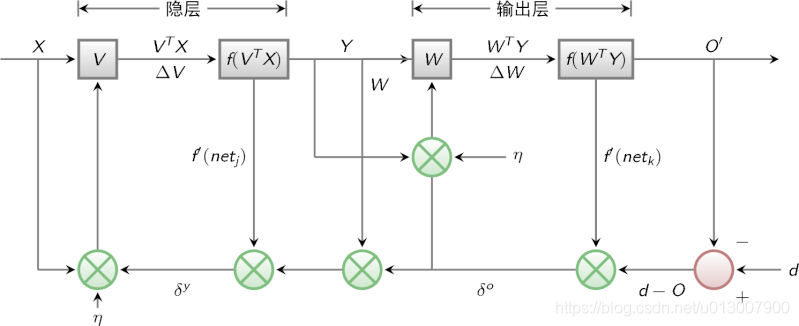3 BP网络特性分析——BP三要素

1)网络拓扑结构；
2)传递函数；
3)学习算法。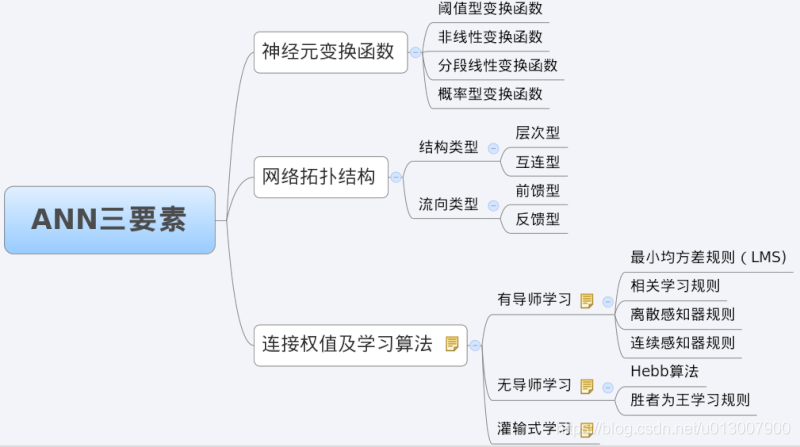3.1 BP网络的拓扑结构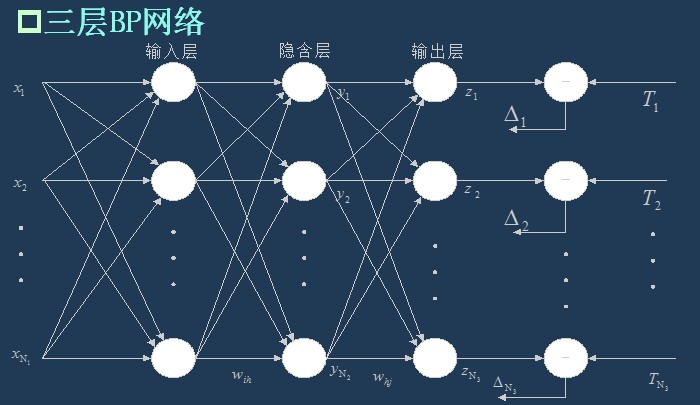3.2 BP网络的传递函数
BP网络采用的传递函数是非线性变换函数——Sigmoid函数（又称S函数）。其特点是函数本身及其导数都是连续的，因而在处理上十分方便。为什么要选择这个函数，等下在介绍BP网络的学习算法的时候会进行进一步的介绍。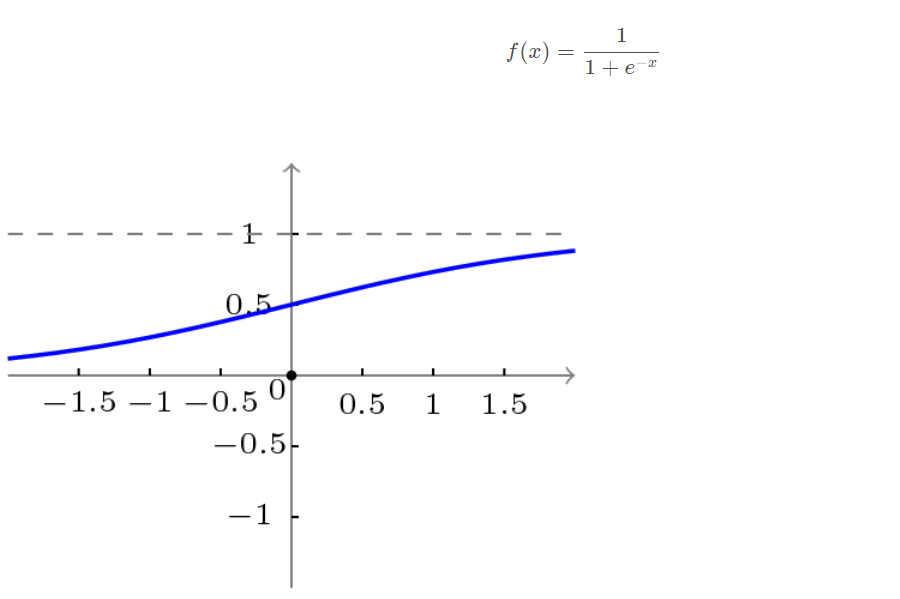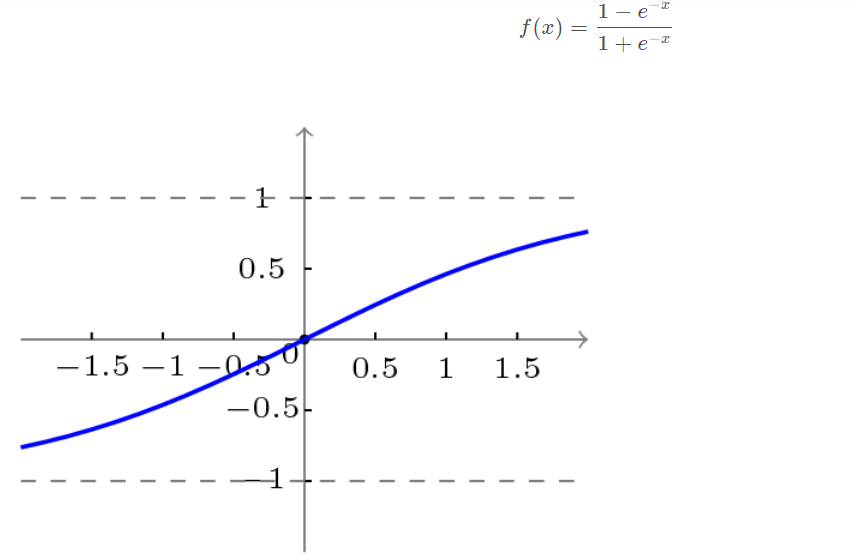3.3 BP网络的学习算法
BP网络的学习算法就是BP算法，又叫 δ 算法（在ANN的学习过程中我们会发现不少具有多个名称的术语）， 以三层感知器为例，当网络输出与期望输出不等时，存在输出误差 E ，定义如下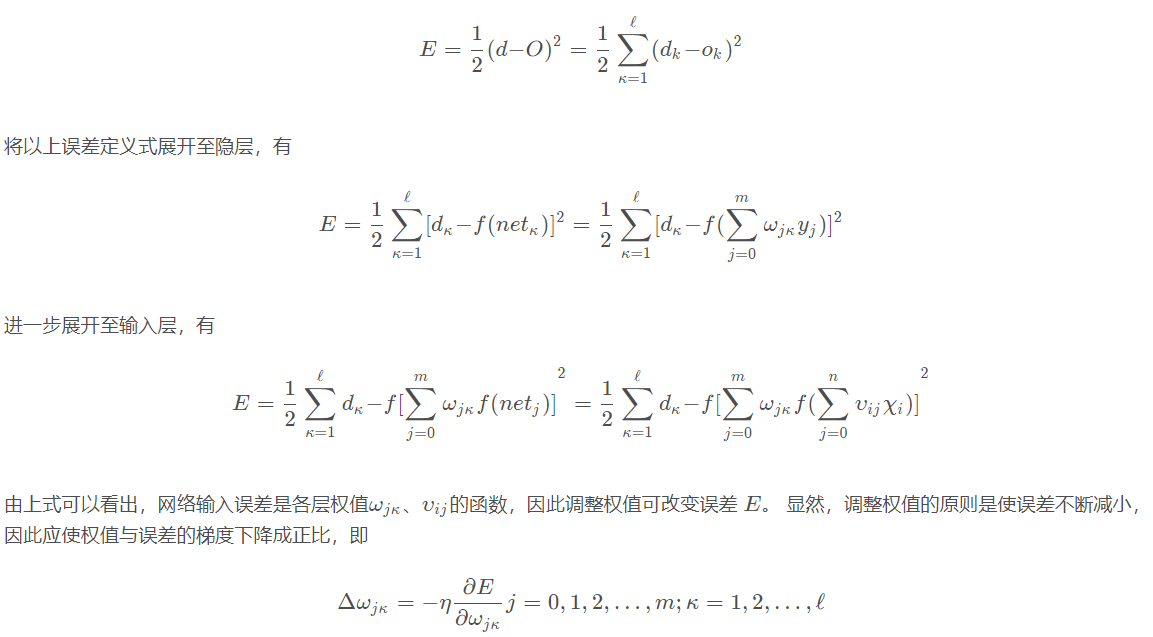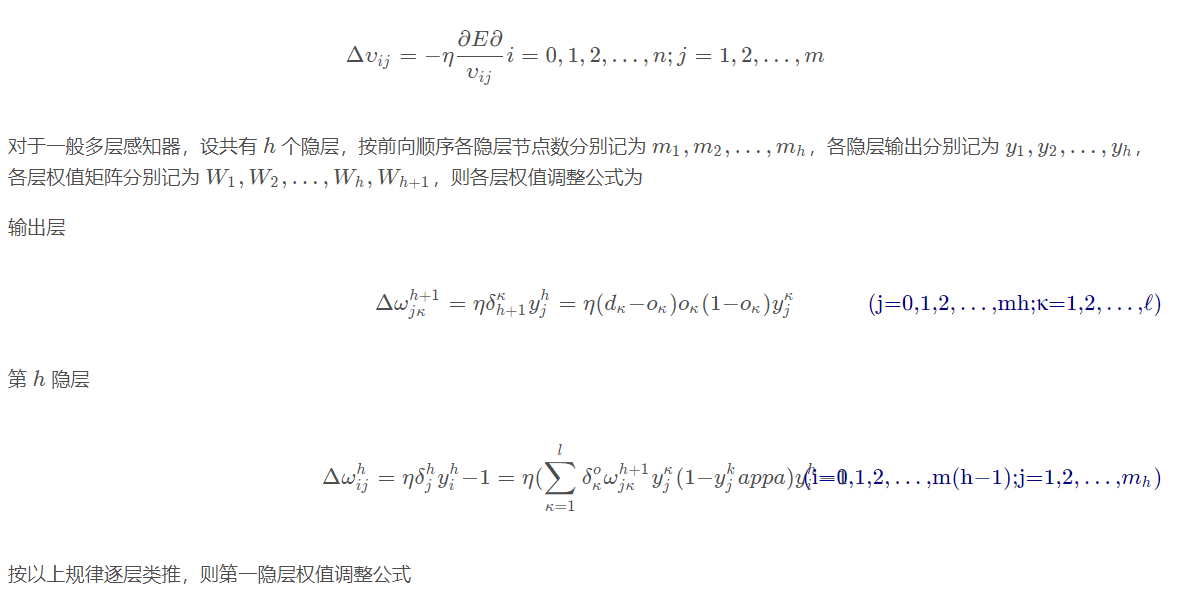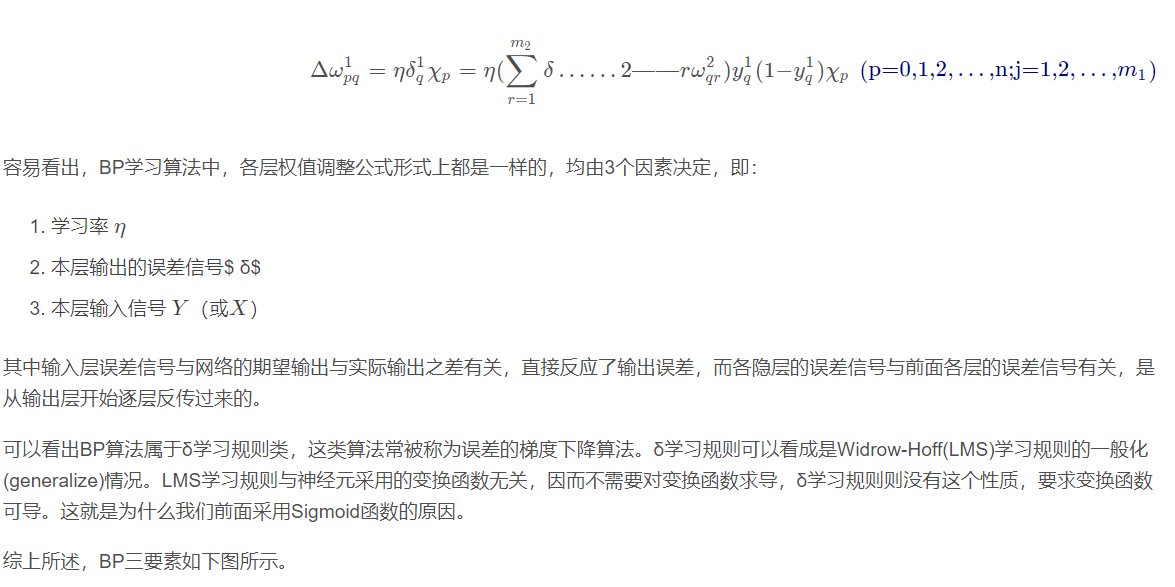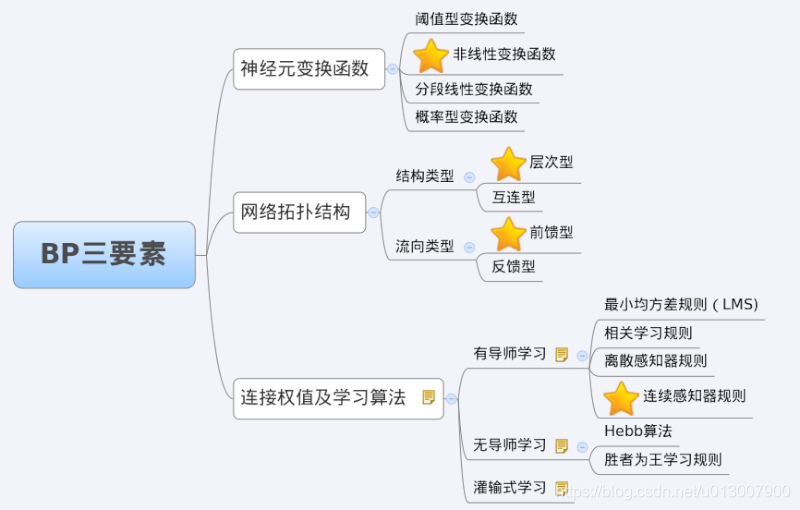4 BP网络的训练分解

``while 终止条件未满足：for record:dataset:trainModel(record)``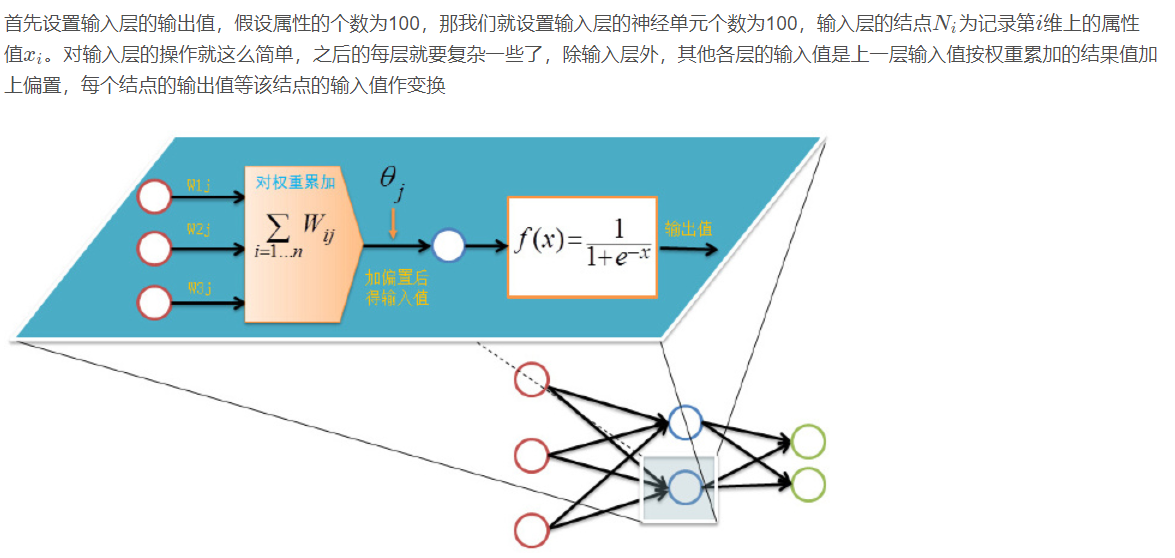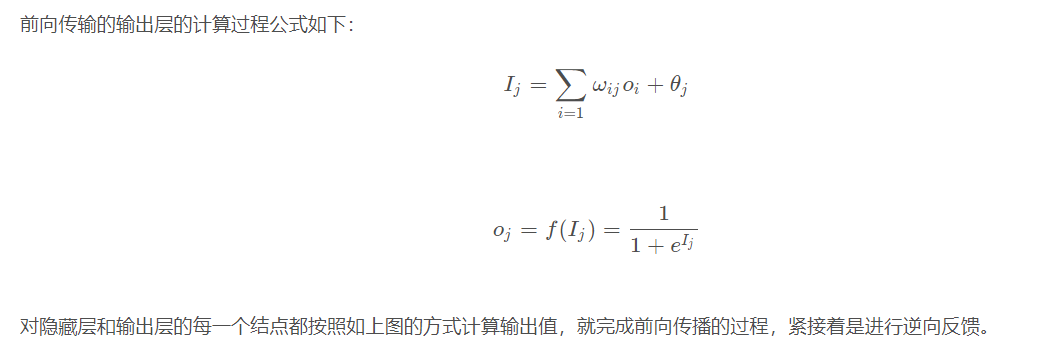4.1 逆向反馈（Backpropagation）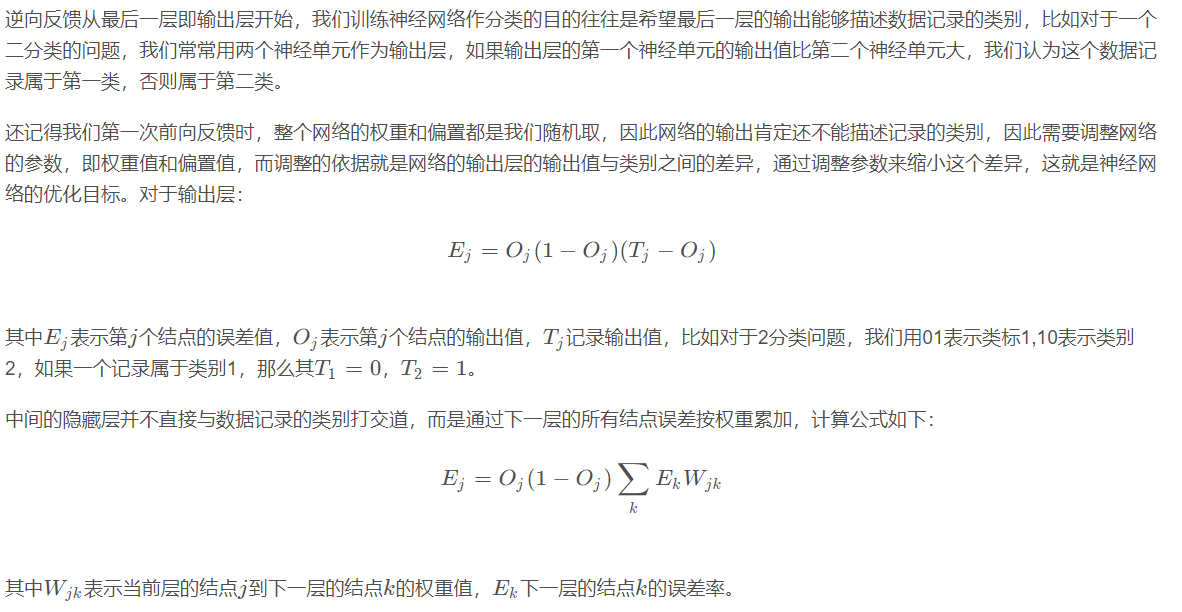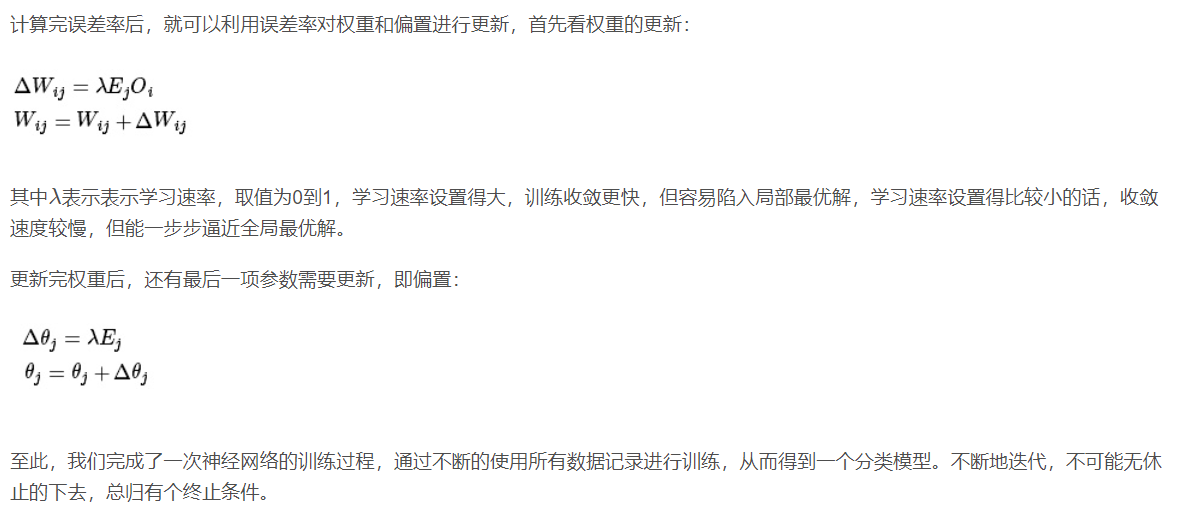4.2 训练终止条件

5 BP网络运行的具体流程
5.1 网络结构

5.2 变量定义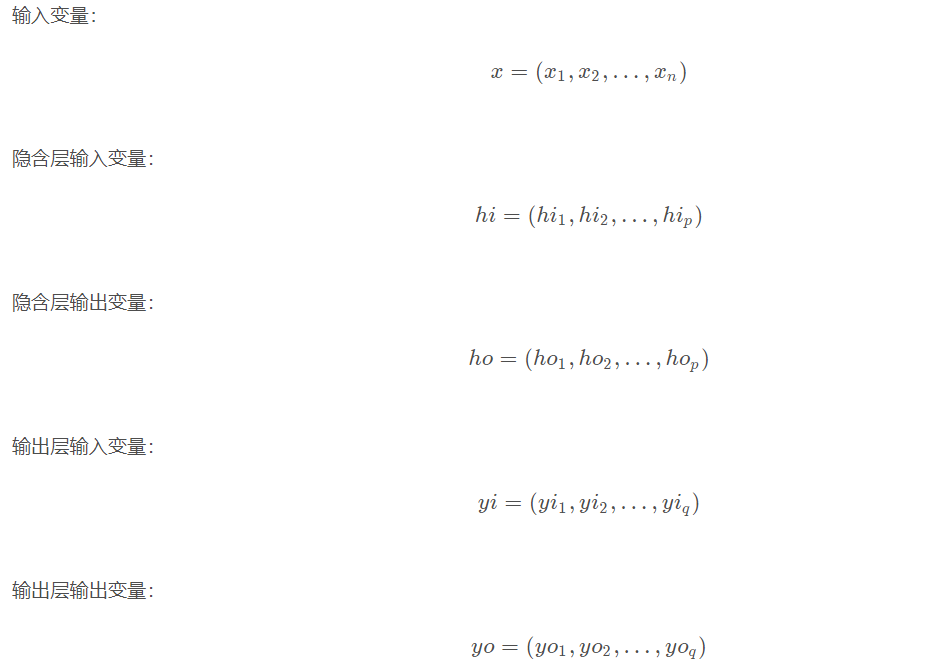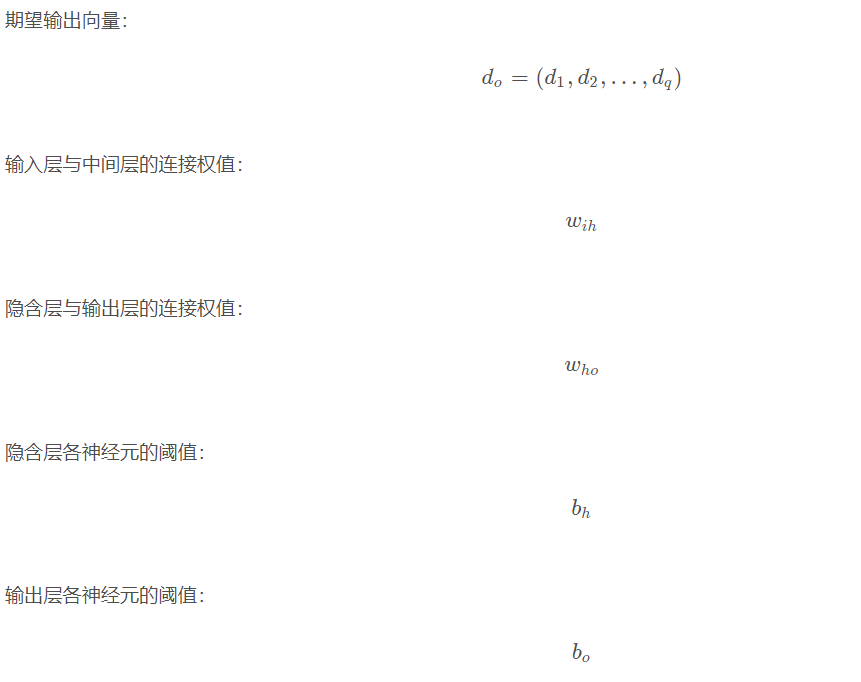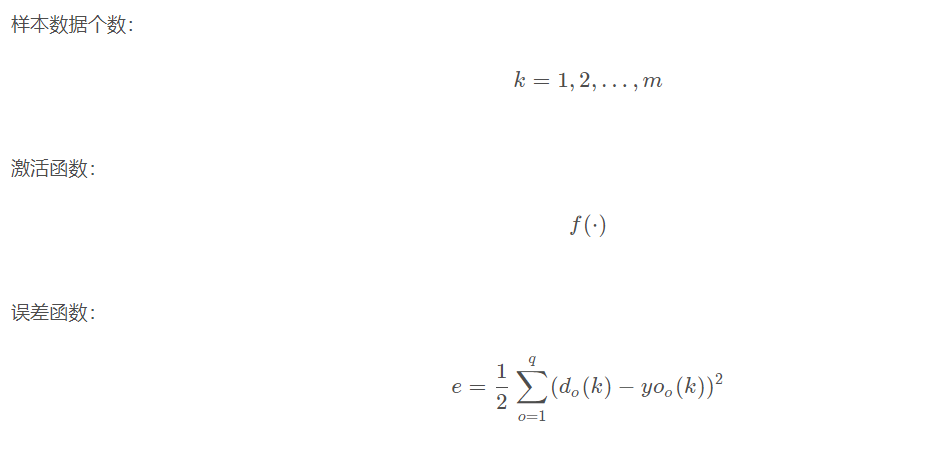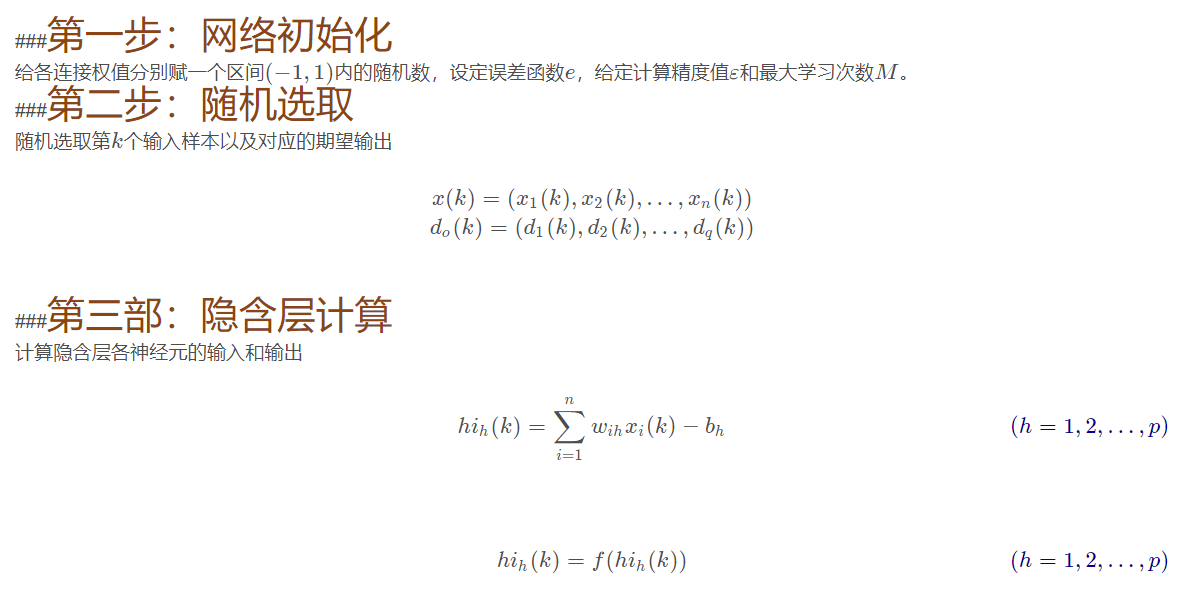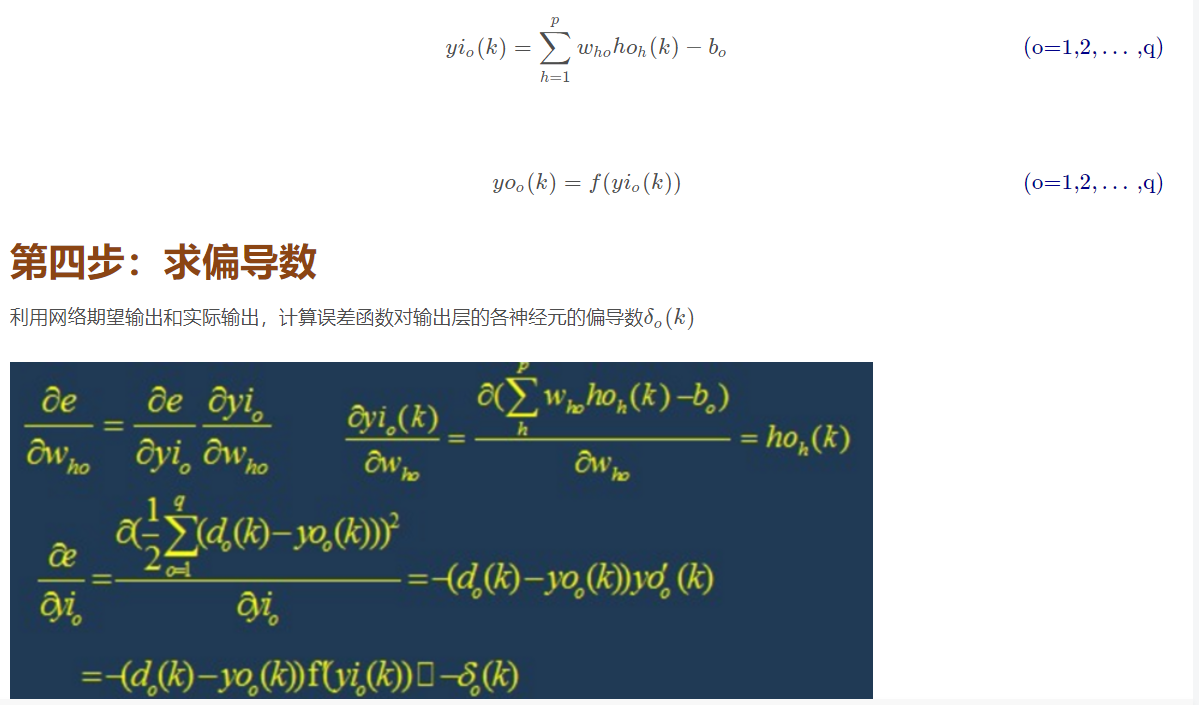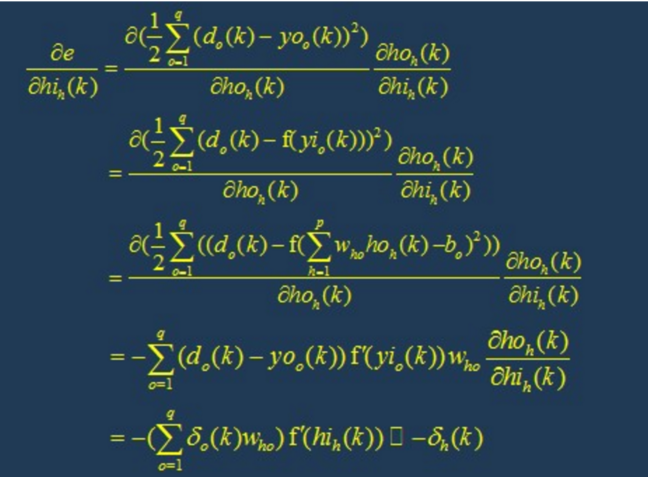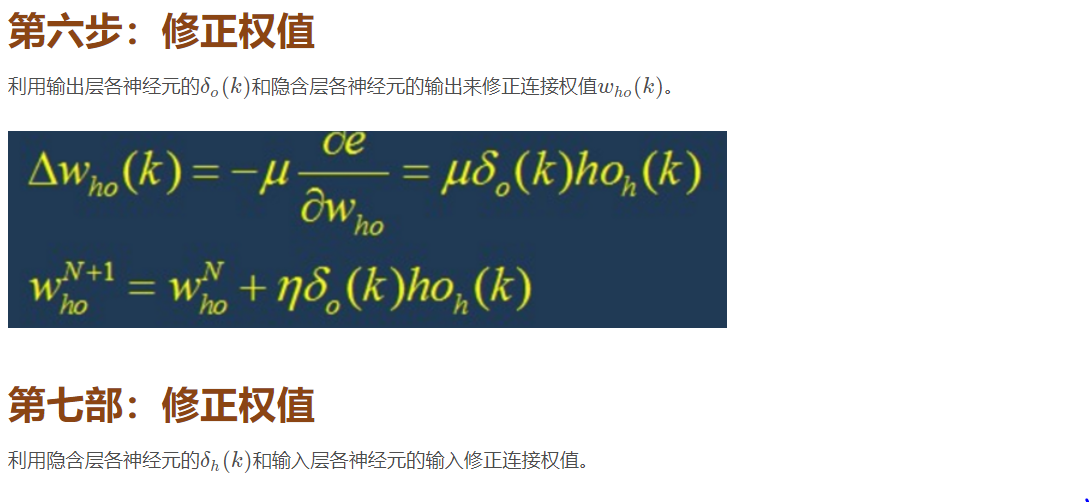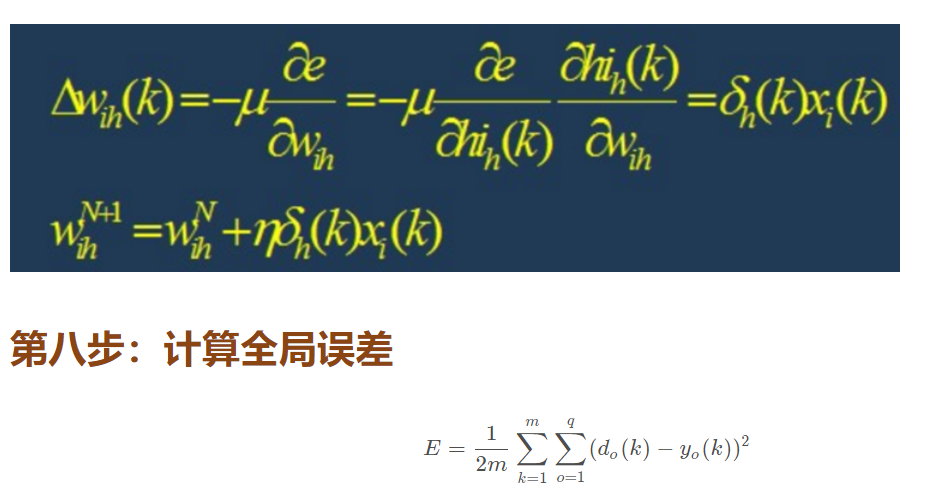6 BP网络的设计

6.1 网络的层数

6.2 隐层神经元的个数

（1）神经元数太少时，网络不能很好的学习，训练迭代的次数也比较多，训练精度也不高。
（2）神经元数太多时，网络的功能越强大，精确度也更高，训练迭代的次数也大，可能会出现过拟合(over fitting)现象。

6.3 初始权值的选取

6.4 学习速率

6.5 期望误差的选取

7 BP网络的局限性
BP网络具有以下的几个问题：

(1)需要较长的训练时间：这主要是由于学习速率太小所造成的，可采用变化的或自适应的学习速率来加以改进。
(2)完全不能训练：这主要表现在网络的麻痹上，通常为了避免这种情况的产生，一是选取较小的初始权值，而是采用较小的学习速率。
(3)局部最小值：这里采用的梯度下降法可能收敛到局部最小值，采用多层网络或较多的神经元，有可能得到更好的结果。

8 BP网络的改进
P算法改进的主要目标是加快训练速度，避免陷入局部极小值等，常见的改进方法有带动量因子算法、自适应学习速率、变化的学习速率以及作用函数后缩法等。 动量因子法的基本思想是在反向传播的基础上，在每一个权值的变化上加上一项正比于前次权值变化的值，并根据反向传播法来产生新的权值变化。而自适应学习 速率的方法则是针对一些特定的问题的。改变学习速率的方法的原则是，若连续几次迭代中，若目标函数对某个权倒数的符号相同，则这个权的学习速率增加， 反之若符号相反则减小它的学习速率。而作用函数后缩法则是将作用函数进行平移，即加上一个常数。

## 二、源代码

``````clc
clear
%--------------------------数据图
figure(1)
plot(data)
xlabel('时间，15min/单位')
ylabel('交通流量/量')%训练数据归一化%%%----------------数据归一化
[datan,dataps]=mapminmax((data)');%-------------------------重构数据
%m=10;
%tau=1;
x=datan;
for t=10:2684
Data(:,(t-9))=[x(t-9) x(t-8) x(t-7) x(t-6) x(t-5) x(t-4) x(t-3) x(t-2) x(t-1) x(t) x(t+1)];
end%--------------------------开始预测
%准备训练数据和测试数据
trn_data = zeros(11,2560);
test_data = zeros(11,100);% prepare training data
trn_data=Data(1:11,1:2560);% prepare checking data
test_data=Data(1:11,2561:2660);%训练数据
input_train=(trn_data(1:10,:));
output_train=(trn_data(11,:));
%测试数据
input_test=(test_data(1:10,:));
output_test=(test_data(11,:));%构建BP神经网络
net=newff(input_train,output_train,[10 1],{'tansig','purelin'},'trainlm');net.trainParam.epochs=7000;  % 训练次数
net.trainParam.goal=0.00001;  % 误差期望值
net.trainParam.lr=0.05;  % 学习率% returns of the train:
%   net--New network
net=train(net,input_train,output_train); %BP神经网络预测输出
y=sim(net,input_test);
%输出结果反归一化
BPoutput=mapminmax('reverse',y,dataps);
output=mapminmax('reverse',output_test,dataps);
%网络预测结果图形
figure(2)
plot(BPoutput,'-r*');   %利用BP产生的预测输出图形
hold on
plot(output,'-b+');  %预测数据的输出图形
legend('预测输出','期望输出')xlabel('时间，15min/单位')
ylabel('交通流量/量')%误差曲线
[DIM_INPUT LEN_DATA ]=size(test_data);  % Data matrix size (1 input vector per row)
for tt=1:LEN_DATA,     error(tt)=abs((BPoutput(tt)-output(tt))/output(tt));ERR(tt)= BPoutput(tt)-output(tt);     % Prediction error
end
``````

## 三、运行结果## 四、备注

http://www.ngui.cc/el/414709.html